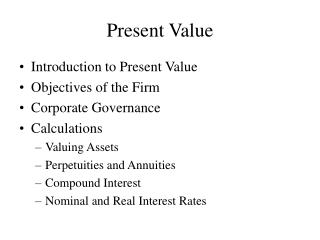DownloadDownload PresentationPresent Value

# Present Value

Télécharger la présentation## Present Value

- - - - - - - - - - - - - - - - - - - - - - - - - - - E N D - - - - - - - - - - - - - - - - - - - - - - - - - - -
##### Presentation Transcript

1. Present Value • Introduction to Present Value • Objectives of the Firm • Corporate Governance • Calculations • Valuing Assets • Perpetuities and Annuities • Compound Interest • Nominal and Real Interest Rates

2. 1 1 + r Introduction to Present Value A dollar now is worth morethan a dollar in the future PV = discount factor x future payment PV = present value Discount factor = r = rate of return to compensate for future (rather than current) payment

3. Ct (1 + rt)t Net Present Value NPV = n t=0  The Net Present Value includes the initial cost of the investment as a cash flow. Net Present Value Rule: Accept investments that have positive net present values

4. Additional Considerations • Risk • Critical, but consideration is deferred until chapter 7 • Rates of Return Return = Profit/Investment Rate of Return Rule: Accept investments that offer rates of return in excess of the opportunity cost of capital The opportunity cost of capital is not the firm’s cost of capital, but the investment’s cost of capital

5. Foundations for Net Present Value Rule • Capital markets and consumption preferences • Financial managers should focus on increasing the market value of the firm • Profit maximization is not sufficient • Timing of profits • Investing in negative NPV projects

6. Corporate Governance • Role of Board of Directors • Incentives to maximizing firm value • Takeovers • Shareholders vs. All Stakeholders • Employees • Customers • Suppliers • Communities

7. Nominal and Real Interest Rates • Nominal interest rates are the actual rates you receive by investing in bank accounts, bonds or other interest bearing securities • Nominal interest rates compensate you both for the time value of money and the erosion of purchasing power caused by inflation • Real interest rates are the returns in excess of the rate of inflation

8. Nominal Interest Rates • Combines inflation and real interest rates 1+i = {(1+q) x (1+r)} where i = nominal interest rate q = inflation rate r = real interest rate • Inflation and nominal interest rates are highly correlated

9. Next Class • Valuation of Bonds and Common Stock • Student presentations • Bond valuation • Stock valuation • Read Chapter 4 in the text